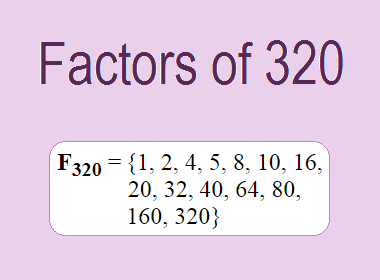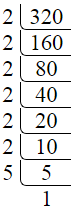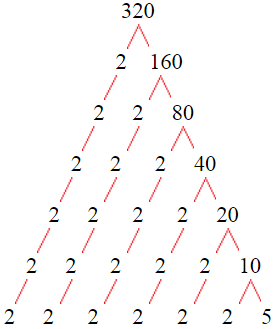# Factors of 320The factors of 320 are 1, 2, 4, 5, 8, 10, 16, 20, 32, 40, 64, 80, 160, and 320 i.e. F320 = {1, 2, 4, 5, 8, 10, 16, 20, 32, 40, 64, 80, 160, 320}. The factors of 320 are all the numbers that can divide 320 without leaving a remainder.

We can check if these numbers are factors of 320 by dividing 320 by each of them. If the result is a whole number, then the number is a factor of 320. Let's do this for each of the numbers listed above:

·        1 is a factor of 320 because 320 divided by 1 is 320.

·        2 is a factor of 320 because 320 divided by 2 is 160.

·        4 is a factor of 320 because 320 divided by 4 is 80.

·        5 is a factor of 320 because 320 divided by 5 is 64.

·        8 is a factor of 320 because 320 divided by 8 is 40.

·        10 is a factor of 320 because 320 divided by 10 is 32.

·        16 is a factor of 320 because 320 divided by 16 is 20.

·        20 is a factor of 320 because 320 divided by 20 is 16.

·        32 is a factor of 320 because 320 divided by 32 is 10.

·        40 is a factor of 320 because 320 divided by 40 is 8.

·        64 is a factor of 320 because 320 divided by 64 is 5.

·        80 is a factor of 320 because 320 divided by 80 is 4.

·        160 is a factor of 320 because 320 divided by 160 is 2.

·        320 is a factor of 320 because 320 divided by 320 is 1.

## How to Find Factors of 320?

1 and the number itself are the factors of every number. So, 1 and 320 are two factors of 320. To find the other factors of 320, we can start by dividing 320 by the numbers between 1 and 320. If we divide 320 by 2, we get a remainder of 0. Therefore, 2 is a factor of 320. If we divide 320 by 3, we get a remainder of 2. Therefore, 3 is not a factor of 320.

Next, we can check if 4 is a factor of 320. If we divide 320 by 4, we get a remainder of 0. Therefore, 4 is also a factor of 320. We can continue this process for all the possible factors of 320.

Through this process, we can find that the factors of 320 are 1, 2, 4, 5, 8, 10, 16, 20, 32, 40, 64, 80, 160, and 320. These are the only numbers that can divide 320 without leaving a remainder.

********************

********************

## Properties of the Factors of 320

The factors of 320 have some interesting properties. One of the properties is that the sum of the factors of 320 is equal to 762. We can see this by adding all the factors of 320 together:

1 + 2 + 4 + 5 + 8 + 10 + 16 + 20 + 32 + 40 + 64 + 80 + 160 + 320 = 762

Another property of the factors of 320 is that the prime factors of 320 are 2, and 5 only.

## Applications of the Factors of 320

The factors of 320 have several applications in mathematics. One of the applications is in finding the highest common factor (HCF) of two or more numbers. The HCF is the largest factor that two or more numbers have in common. For example, to find the HCF of 320 and 120, we need to find the factors of both numbers and identify the largest factor they have in common. The factors of 320 are 1, 2, 4, 5, 8, 10, 16, 20, 32, 40, 64, 80, 160, and 320. The factors of 120 are 1, 2, 3, 5, 6, 8, 10, 12, 15, 20, 24, 30, 40, 60, and 120. The largest factor that they have in common is 40. Therefore, the HCF of 320 and 120 is 40.

Another application of the factors of 320 is in prime factorization. Prime factorization is the process of expressing a number as the product of its prime factors. The prime factors of 320 are 2, and 5, since these are the only prime numbers that can divide 320 without leaving a remainder. Therefore, we can express 320 as:

320 = 2 × 2 × 2 × 2 × 2 × 2 × 5

We can do prime factorization by division and factor tree method also. Here is the prime factorization of 320 by division method,320 = 2 × 2 × 2 × 2 × 2 × 2 × 5

Here is the prime factorization of 320 by the factor tree method,320 = 2 × 2 × 2 × 2 × 2 × 2 × 5

## Conclusion

The factors of 320 are the numbers that can divide 320 without leaving a remainder. The factors of 320 are 1, 2, 4, 5, 8, 10, 16, 20, 32, 40, 64, 80, 160, and 320. The factors of 320 have some interesting properties, such as having a sum of 762. The factors of 320 have several applications in mathematics, such as finding the highest common factor and prime factorization.